# XGBoost for Regression

The results of the regression problems are continuous or real values. Some commonly used regression algorithms are Linear Regression and Decision Trees. There are several metrics involved in regression like root-mean-squared error (RMSE) and mean-squared-error (MAE). These are some key members for XGBoost models, each plays their important roles.

• RMSE: It is the square root of mean squared error (MSE).
• MAE: It is an absolute sum of actual and predicted differences, but it lacks mathematically, that’s why it is rarely used, as compared to other metrics.

XGBoost is a powerful approach for building supervised regression models. The validity of this statement can be inferred by knowing about its (XGBoost) objective function and base learners.

The objective function contains loss function and a regularization term. It tells about the difference between actual values and predicted values, i.e how far the model results are from the real values. The most common loss functions in XGBoost for regression problems is `reg:linear`, and that for binary classification is `reg:logistics`.

Ensemble learning involves training and combining individual models (known as base learners) to get a single prediction, and XGBoost is one of the ensemble learning methods. XGBoost expects to have the base learners which are uniformly bad at the remainder so that when all the predictions are combined, bad predictions cancels out and better one sums up to form final good predictions.

Code:

 `# Necessary imports ` `import` `numpy as np ` `import` `pandas as pd ` `import` `xgboost as xg ` `from` `sklearn.model_selection ``import` `train_test_split ` `from` `sklearn.metrics ``import` `mean_squared_error as MSE ` ` `  `# Load the data ` `dataset ``=` `pd.read_csv(``"boston_house.csv"``) ` `X, y ``=` `dataset.iloc[:, :``-``1``], dataset.iloc[:, ``-``1``] ` ` `  `# Splitting ` `train_X, test_X, train_y, test_y ``=` `train_test_split(X, y, ` `                      ``test_size ``=` `0.3``, random_state ``=` `123``) ` ` `  `# Instantiation ` `xgb_r ``=` `xg.XGBRegressor(objective ``=``'reg:linear'``, ` `                  ``n_estimators ``=` `10``, seed ``=` `123``) ` ` `  `# Fitting the model ` `xgb_r.fit(train_X, train_y) ` ` `  `# Predict the model ` `pred ``=` `xgb_r.predict(test_X) ` ` `  `# RMSE Computation ` `rmse ``=` `np.sqrt(MSE(test_y, pred)) ` `print``(``"RMSE : % f"` `%``(rmse)) `

Output:

```129043.2314
```

Code: Linear base learner

 `# Necessary imports ` `import` `numpy as np ` `import` `pandas as pd ` `import` `xgboost as xg ` `from` `sklearn.model_selection ``import` `train_test_split ` `from` `sklearn.metrics ``import` `mean_squared_error as MSE ` ` `  `# Load the data ` `dataset ``=` `pd.read_csv(``"boston_house.csv"``) ` `X, y ``=` `dataset.iloc[:, :``-``1``], dataset.iloc[:, ``-``1``] ` ` `  `# Splitting ` `train_X, test_X, train_y, test_y ``=` `train_test_split(X, y,  ` `                      ``test_size ``=` `0.3``, random_state ``=` `123``) ` ` `  `# Train and test set are converted to DMatrix objects, ` `# as it is required by learning API. ` `train_dmatrix ``=` `xg.DMatrix(data ``=` `train_X, label ``=` `train_y) ` `test_dmatrix ``=` `xg.DMatrix(data ``=` `test_X, label ``=` `test_y) ` ` `  `# Parameter dictionary specifying base learner ` `param ``=` `{``"booster"``:``"gblinear"``, ``"objective"``:``"reg:linear"``} ` ` `  `xgb_r ``=` `xg.train(params ``=` `param, dtrain ``=` `train_dmatrix, num_boost_round ``=` `10``) ` `pred ``=` `xgb_r.predict(test_dmatrix) ` ` `  `# RMSE Computation ` `rmse ``=` `np.sqrt(MSE(test_y, pred)) ` `print``(``"RMSE : % f"` `%``(rmse)) `

Output:

``` 124326.24465
```

Note: The dataset needs to be converted into DMatrix. It is an optimized data structure that the creators of XGBoost made. It gives the package its performance and efficiency gains.

The loss function is also responsible for analyzing the complexity of the model, and it the model becomes more complex there becomes a need to penalize it and this can be done using Regularization. It penalizes more complex models through both LASSO (L1) and Ridge (L2) regularization to prevent overfitting. The ultimate goal is to find simple and accurate models.

Regularization parameters are as follows:

• gamma: minimum reduction of loss allowed for a split to occur. Higher the gamma, fewer the splits.
alpha: L1 regularization on leaf weights, larger the value, more will be the regularization, which causes many leaf weights in the base learner to go to 0.
• lamba: L2 regularization on leaf weights, this is smoother than L1 nd causes leaf weights to smoothly decrease, unlike L1, which enforces strong constraints on leaf weights.

Below are the formulas which help in building the XGBoost tree for Regression.

Step 1: Calculate the similarity scores, it helps in growing the tree.

```Similarity Score = (Sum of residuals)^2 / Number of residuals + lambda
```

Step 2: Calculate the gain to determine how to split the data.

```Gain = Left tree (similarity score) + Right (similarity score) - Root (similarity score)
```

Step 3: Prune the tree by calculating the difference between Gain and gamma (user-defined tree-complexity parameter)

```Gain - gamma
```

If the result is a positive number then do not prune and if the result is negative, then prune and again subtract gamma from the next Gain value way up the tree.

Step 4: Calculate output value for the remaining leaves

```Output value = Sum of residuals / Number of residuals + lambda
```

Note: If the value of lambda is greater than 0, it results in more pruning by shrinking the similarity scores and it results in smaller output values for the leaves.

Let’s see a part of mathematics involved in finding the suitable output value to minimize the loss function

For classification and regression, XGBoost starts with an initial prediction usually 0.5, as shown in the below diagram.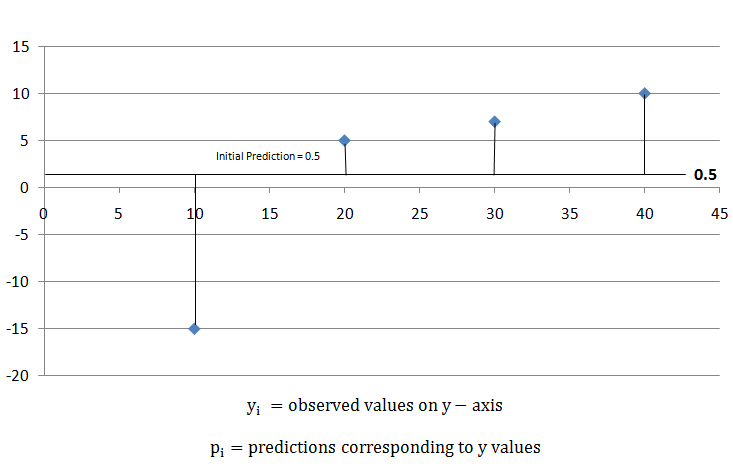To find how good the prediction is, calculate the Loss function, by using the formula,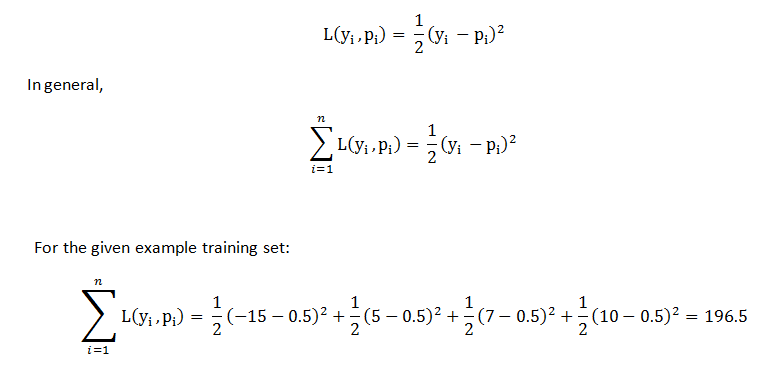For the given example, it came out to be 196.5. Later, we can apply this loss function and compare the results, and check if predictions are improving or not.

XGBoost uses those loss function to build trees by minimizing the below equation: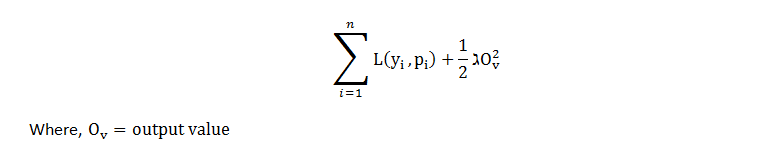The first part of the equation is the loss function and the second part of the equation is the regularization term and the ultimate goal is to minimize the whole equation.

For optimizing output value for the first tree, we write the equation as follows, replace p(i) with the initial predictions and output value and let lambda = 0 for simpler calculations. Now the equation looks like,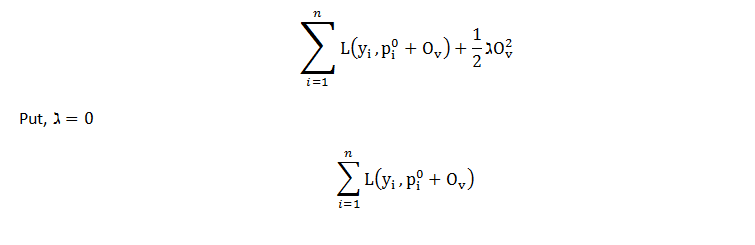The loss function for initial prediction was calculated before, which came out to be 196.5.

So, for output value = 0, loss function = 196.5. Similarly, if we plot the point for output value = -1, loss function = 203.5 and for output value = +1, loss function = 193.5, and so on for other output values and, if we plot this in the graph. we get a parabola like structure. This is the plot for the equation as a function of output values.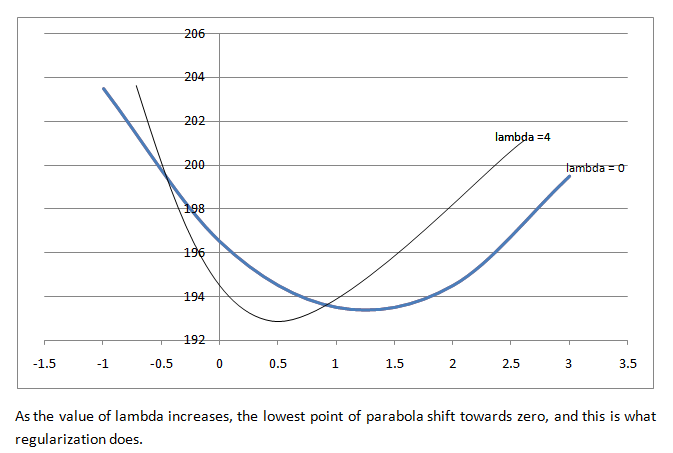If lambda = 0, the optimal output value is at the bottom of the parabola where the derivative is zero. XGBoost uses Second-Order Taylor Approximation for both classification and regression. The loss function containing output values can be approximated as follows:The first part is Loss Function, the second part includes the first derivative of the loss function and the third part includes the second derivative of the loss function. The first derivative is related o Gradient Descent, so here XGBoost uses ‘g’ to represent the first derivative and the second derivative is related to Hessian, so it is represented by ‘h’ in XGBoost. Plugging the same in the equation: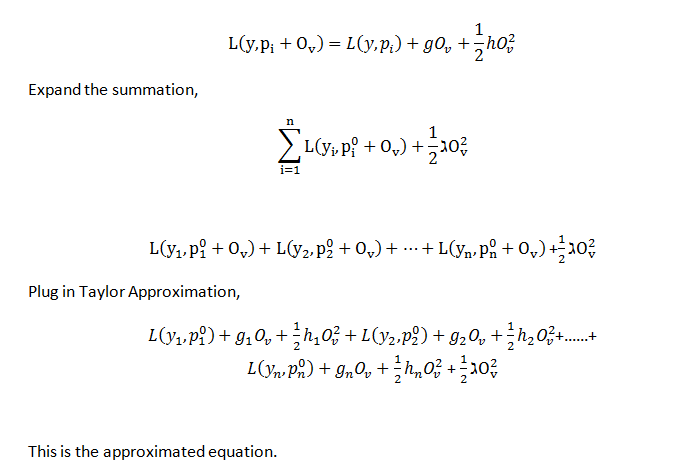Remove the terms that do not contain the output value term, now minimize the remaining function by following steps:

• Take the derivative w.r.t output value.
• Set derivative equals 0 (solving for the lowest point in parabola)
• Solve for the output value.
• g(i) = negative residuals
• h(i) = number of residuals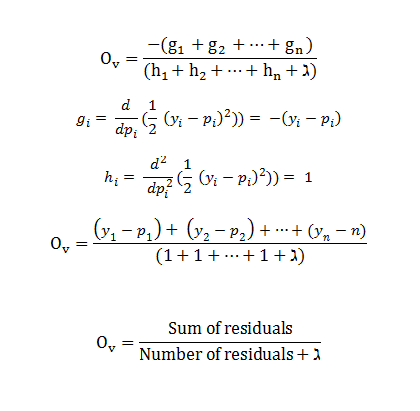This is the output value formula for XGBoost in Regression. It gives the x-axis coordinate for the lowest point in the parabola.

Attention geek! Strengthen your foundations with the Python Programming Foundation Course and learn the basics.

To begin with, your interview preparations Enhance your Data Structures concepts with the Python DS Course.

My Personal Notes arrow_drop_upCheck out this Author's contributed articles.

If you like GeeksforGeeks and would like to contribute, you can also write an article using contribute.geeksforgeeks.org or mail your article to contribute@geeksforgeeks.org. See your article appearing on the GeeksforGeeks main page and help other Geeks.

Please Improve this article if you find anything incorrect by clicking on the "Improve Article" button below.